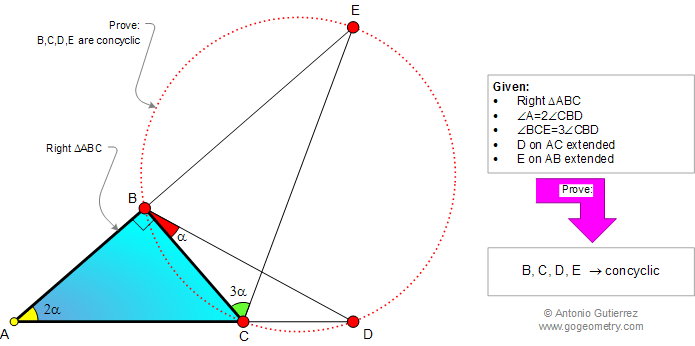# Online Geometry Problem 911: Right Triangle, Double Angle, Triple Angle, Concyclic Points, Cyclic Quadrilateral. Level: High School, College, Mathematics Education

< PREVIOUS PROBLEM  |  NEXT PROBLEM >

 The figure shows a right triangle ABC, D is on AC extended and E on AB extended, angle A = 2(angle CBD), and angle BCE = 3(angle CBD). Prove that the points B, C, D, and E are concyclic ((lie on a same circle).Geometry problem solving is one of the most challenging skills for students to learn. When a problem requires auxiliary construction, the difficulty of the problem increases drastically, perhaps because deciding which construction to make is an ill-structured problem. By “construction,” we mean adding geometric figures (points, lines, planes) to a problem figure that wasn’t mentioned as "given."
 Home | Search | Geometry | Problems | All Problems | Open Problems | Visual Index | 10 Problems | Problems Art Gallery |  Art | 911-920 | Triangles | Right Triangle | Double Angle | Cyclic Quadrilateral | Concyclic Points | by Antonio Gutierrez